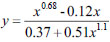Table 1: Guo’s compressive stress-strain relationship of confined concrete (Guo & Shi (2003)).

Confinement Factor λt≤0.32 λt>0.32
Peak stress fc,c=(1+0.5λt)fc fc,c=(0.55+1.9λt)fc
Strain at peak stress εpc=(1+2.5λt)εp εpc=(-6.2+25λt)εp
Curve functions
x=ε/εpc
y=σ/fc,c
y=αa,cx+(3-2αa,c)x2+(αa,c-2)x3(x≤1.0)y=x/(αd,c(x-1)2+x)(x>1.0)